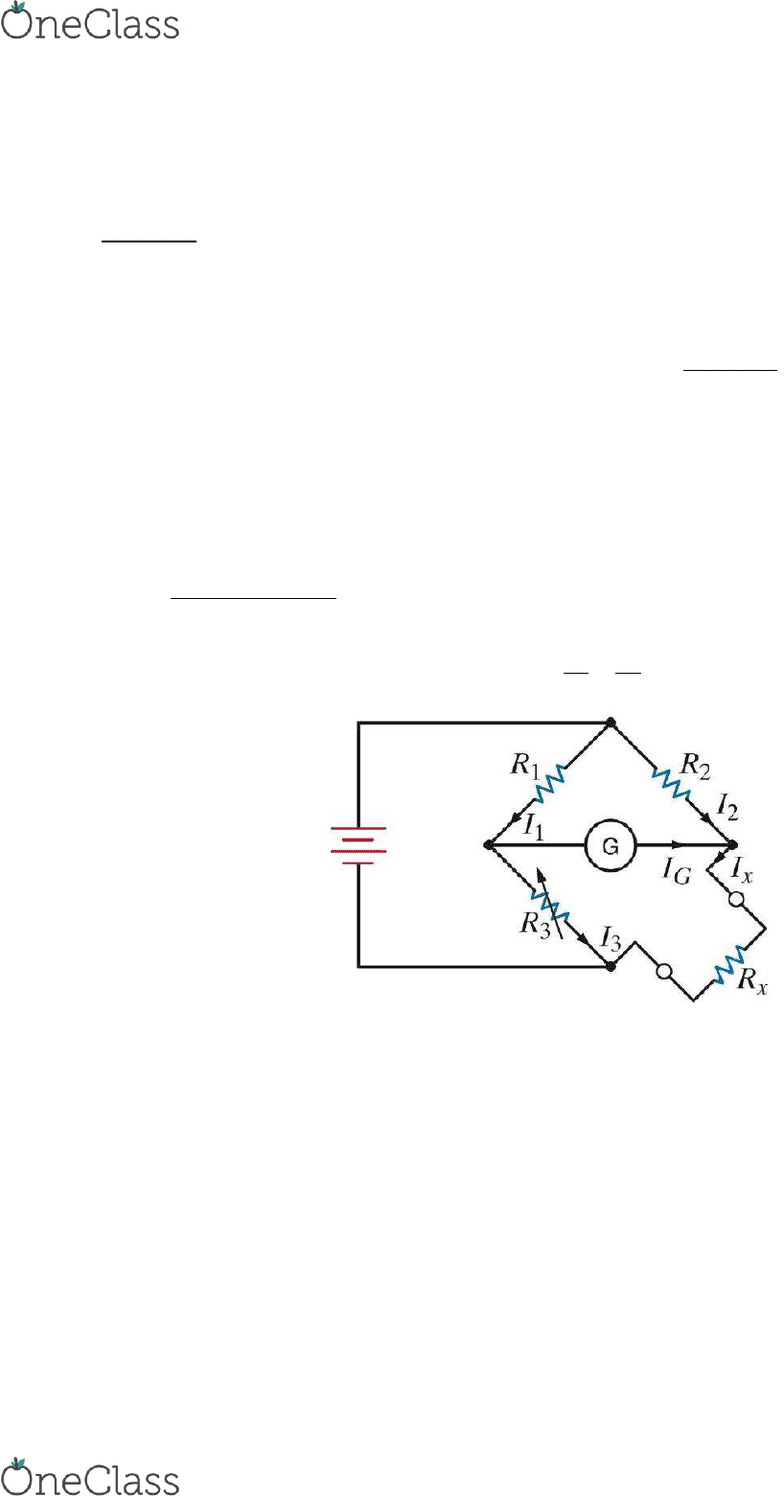Textbook Notes (290,000)
CA (170,000)
UBC (3,000)
EECE (3)
S T T (1)
Chapter 2, 3, 6

# EECE 265 Chapter Notes - Chapter 2, 3, 6: Charles Wheatstone, Short Circuit, Capacitor

Department
Electrical and Computer Engineering
Course Code
EECE 265
Professor
S T T
Chapter
2, 3, 6

This preview shows page 1. to view the full 4 pages of the document.EECE 263 Course Notes
Chapter 2 Resistive Circuit
Basic Stuff:
V = R * I
V = I / G ; G = 1/R
- Short circuit: at 0 resistance, conductance is infinity making voltage = 0
- Ope iruit: 0 odutae, ires are ot oeted lol….
P = V*I
P = V^2/R
P = R*I^2
- Power is instantaneous; but the equations are used in a way to reflect long term steady state
- Wheatstone Bridge measures resistance; adjusts R3 until current in G is 0 so a simple balanced
relationship can be used to find Rx:




- True Node 3+ elements connected to it (incl. interconnection wires)
- Binary Node 2 elements connected to it
- Branch connection between 2 nodes (incl. all elements in it)
- Loop closed circuit path; cross true nodes only once
find more resources at oneclass.com
find more resources at oneclass.com